## Cascaded Conditional Region Variable Assignments

In R2021b, HDL Coder™ provides a coding standard (Guideline 2.F.B.1.a in RTL Description Rules and Checks) to check for assignments to the same variable in multiple cascaded control regions within the same process block. HDL Coder points to the blocks that generate such a coding style. If the style is not recommended for use in your production workflow, consider an alternative coding style or replace the block pointed to by the rule. The check displays an error if the generated HDL code for your design contains the same variable/signal for `VHDL` or register/wire for `Verilog` written to in multiple cascaded conditional regions, such as `switch case` or `if/else` cascaded regions, within the same process block. To turn on this check for your model, see Check for assignments to the same variable in multiple cascaded control regions.

### Example Patterns that Fail the Check for Guideline 2.F.B.1.a

These examples show HDL Code patterns that fail the check for the presence of assignments to the same variable in multiple cascaded conditional regions.

```always @(u) begin if(u < 8'sd4) begin y = -8'sd1; end if(u == 8'sd1) begin y = 8'sd10; else y =8'sd2; end end```

#### Parallel Cascaded 'if' Regions with Nesting

```always @(u, k) begin​ if (u < 8’sd4) begin​ if(k == 8’sd1) begin ​ y = 8'sd10;​ end​ end​ ​ if (u < 8’sd2) begin​ y = 8'sd1;​ else​ y = 8’sd2;​ end​ end​```

#### Parallel Cascaded 'if' and 'switch' Regions

```always @(u) begin​ if (u < 8’sd4) begin​ y = 8'sd10;​ end​ case (u)​ 8’sd1:​ y = 8’sd11;​ default:​ y = 8’sd4;​ endcase​ end​```

```always @(u) begin​ case (u)​ 8’sd2:​ y = 8’sd15;​ default:​ y = 8’sd4;​ endcase​ case (u)​ 8’sd1:​ y = 8’sd11;​ default:​ y = 8’sd4;​ endcase​ end​```

#### Parallel Cascaded Region Inside Nested Region

```always @(u) begin​ case (u)​ 8’sd2:​ y = 8’sd15;​ default:​ if (u < 8’sd4) begin​ y = 8'sd10;​ end​ if (u == 8’sd1) begin​ y = 8'sd10;​ else​ y = 8’sd2;​ end​ endcase​ end​```

### Example Patterns that Pass the Check for Guideline 2.F.B.1.a

These examples show HDL Code patterns that pass the check for the presence of assignments to the same variable in multiple cascaded conditional regions if you enable the check.

#### Default Value and Conditional Assignment

```always @(u) begin​ y = 8’sd1;​ if (u < 8’sd4) begin​ y = 8'sd10;​ end​ end​```

#### Parallel cascaded “if” Regions Writing to Different Outputs​

```always @(u) begin​ if (u < 8’sd4) begin​ y1 = -8'sd1;​ else​ y1 = 8’sd3;​ end​ ​ if (u == 8’sd1) begin​ y2 = 8'sd10;​ else​ y2 = 8’sd2;​ end​ end​```

#### Assignment in All Paths of Condition Regions​

```always @(u) begin​ if (u == 8’sd1) begin​ y = 8'sd10;​ else​ y = 8’sd2;​ end​ end​```

### Simulink Blocks and Modeling Patterns that Fail the Check for Guideline 2.F.B.1.a

Some Simulink blocks and modeling patterns can fail this check (guideline 2.F.B.1.a) and cause an error during HDL code generation. If a block or modeling pattern you use in your code fails this check , consider disabling the check if it is not needed in your production workflow or changing the block or modeling pattern producing the code failure.

#### MATLAB Function Block

In some cases, MATLAB® code written inside MATLAB Function blocks might generate HDL Code that fails this check.

Conditional Initialization of Persistent Variables.  If the persistent variables in a MATLAB Function block are conditionally initialized and then overwritten in a conditional region elsewhere in the code, the generated code might fail this check.

For example, if you have a MATLAB Function block in your DUT with the MATLAB code:

```function y = fcn(u) persistent loc; if(isempty(loc) || u == int8(2)) loc = int8(-1); end % persistent variable overwritten in conditional code region if(u<int8(4)) loc = int8(10); end y = loc;```

This Verilog code snippet demonstrates the violation of a value assignment to the variable loc_temp in two parallel conditional `if` statements.

```always @(loc, loc_not_empty, u) begin loc_temp = loc; loc_not_empty_next = loc_not_empty; if ( ! loc_not_empty || (u == 8'sd2)) begin loc_temp = -8'sd1; loc_not_empty_next = 1'b1; end // persistent variable overwritten in conditional code region if (u < 8'sd4) begin loc_temp = 8'sd10; end ... end```

Explicit modeling in the MATLAB Function block.  If you set the HDL Architecture in the HDL Block Properties dialog box of the MATLAB Function block as `MATLAB Function` and design your MATLAB code in a coding style that assigns multiple values to the same variable in cascading conditional regions, an error might occur from the resulting violating patterns in the generated HDL code.

For example, if you have a MATLAB Function block in your DUT with the MATLAB code:

```function y = fcn(u) % if region 1 if(u<4) % if region 1-1 if(u==1) y = int8(0); else y = int8(4); end % if region 1-2 if(u==2) y = int8(5); end else y = int8(6); end ... end```

Region 1 in the preceding code contains two `if` statements that assign different values to the same output variable y. This pattern causes a violation in the generated Verilog code.

```module mlfb (u, y); ... always @(u) begin // if region 1 if (u < 8'sd4) begin // if region 1-1 if (u == 8'sd1) begin y_1 = 8'sd0; end else begin y_1 = 8'sd4; end // if region 1-2 if (u == 8'sd2) begin y_1 = 8'sd5; end end else begin y_1 = 8'sd6; end ... end assign y = y_1; endmodule // mlfb```

Conditional Assignments of Matrix Variables.  If you assign different indices of a single matrix in multiple cascading conditional regions in a MATLAB Function block, a violation can occur.

For example, if you have a MATLAB Function block in your DUT with the MATLAB code:

```function y = fcn(u) y = int8(zeros(1,2)); % if region 1 if(u==1) y(1) = int8(2); end % if region 2 if(u==2) y(2) = int8(5); end```

Although two different indices of the matrix y are being assigned values in cascading `if` statements, this code generates Verilog code that causes a violation.

```ARCHITECTURE rtl OF mlfb IS -- Signals SIGNAL u_signed : signed(7 DOWNTO 0); -- int8 SIGNAL y_tmp : vector_of_signed8(0 TO 1); -- int8  BEGIN u_signed <= signed(u); mlfb_1_output : PROCESS (u_signed) BEGIN FOR t_0 IN 0 TO 1 LOOP y_tmp(t_0) <= to_signed(16#00#, 8); END LOOP; -- if region 1 IF u_signed = to_signed(16#00000001#, 8) THEN y_tmp(0) <= to_signed(16#02#, 8); END IF; -- if region 2 IF u_signed = to_signed(16#00000002#, 8) THEN y_tmp(1) <= to_signed(16#05#, 8); END IF; END PROCESS mlfb_1_output; outputgen: FOR k IN 0 TO 1 GENERATE y(k) <= std_logic_vector(y_tmp(k)); END GENERATE; END rtl;```

Workarounds

• Design MATLAB code in your MATLAB Function block in a way that avoids patterns that assign multiple values to the same variable in multiple cascaded conditional regions.

• Consider specifying the HDL Block property of the MATLAB Function block HDL Architecture to be ```MATLAB Datapath```.

#### Stateflow Charts

In some cases, MATLAB code written in Stateflow® chart can generate HDL code that violates this check.

Explicit Chart Patterns.  Cascaded `if` regions containing assignments to the same variable in a Stateflow chart can produce the equivalent pattern in the generated HDL code.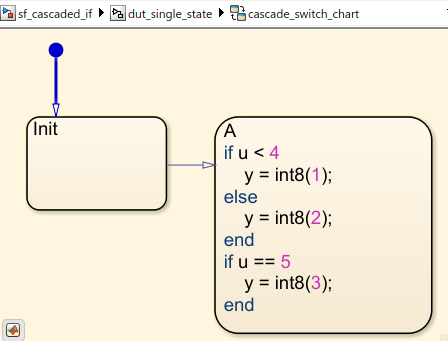One `if` region in a transition followed by another `if` region in a destination state can cause a violation in the generated HDL code. This pattern is seen in this Stateflow Chart: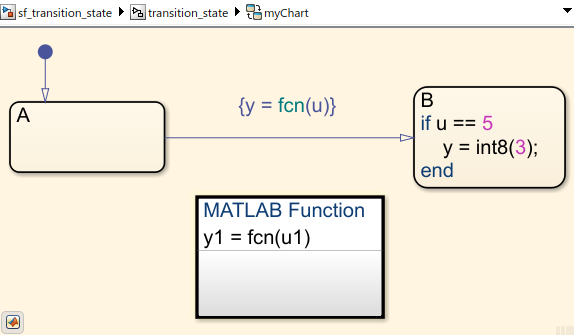With the MATLAB Function block in the transition containing the code:

```function y1 = fcn(u1) if(u1<4) y1 = int8(5); else y1 = int8(6); end end```

The workaround for both of these explicit chart pattern violations is to change the design pattern used in creating the Stateflow charts. Avoid using explicit `if/else` or `switch/case` regions in your Stateflow charts. Instead, redesign the required control flows by using Stateflow chart semantics, such as transitions and states.

Parallel Decomposition.  In Stateflow charts, you have an option to choose Parallel (AND) mode for the Decomposition property of the chart that changes the chart execution to parallel style. For more information, see Execution Order for Parallel States (Stateflow).

For example, when you specify parallel decomposition in a chart, it results in the generation of cascaded `switch` regions in the same `always` process.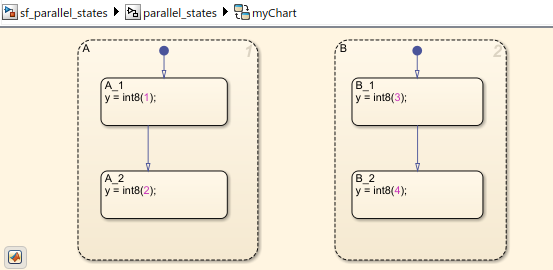If the same output variable is assigned a value in multiple parallel states, a violation occurs in the generated HDL code. This Verilog code snippet demonstrates the violation of a value assignment to the variable y_1 in two parallel conditional `case` statements in the same `always` process, generated as a result of parallel decomposition.

```always @(is_A, is_B) begin is_A_next = is_A; is_B_next = is_B; case ( is_A) state_type_is_A_IN_A_1 : begin is_A_next = state_type_is_A_IN_A_2; y_1 = 8'sd2; end default : begin //case IN_A_2: y_1 = 8'sd2; end endcase case ( is_B) state_type_is_B_IN_B_1 : begin is_B_next = state_type_is_B_IN_B_2; y_1 = 8'sd4; end default : begin //case IN_B_2: y_1 = 8'sd4; end endcase end```

Temporal Logic.  Use of temporal logic in your Stateflow chart generally results in violations. Under some conditions, generated code does not violate the coding guideline. Avoid using temporal logic to ensure that the generated code does not result in a violation.

#### LUT-Based Sine, Cosine Block

When using the LUT-based Sine, Cosine block in your model to generate HDL code, a violation of this check might occur in the generated HDL code. This Verilog code snippet generated from a Simulink model containing a LUT-based Sine, Cosine block demonstrates the violation of a value assignment to the variable Look_Up_Table_k in multiple parallel conditional `if` statements.

```always @(QuadHandle2_out1) begin Look_Up_Table_t_0_0 = 16'sb0000000000000000; ... Look_Up_Table_t_0_0 = 16'sb0011111111101100; Look_Up_Table_t_0_0 = 16'sb0100000000000000; Look_Up_Table_in0_0 = 18'sb000000000000000000; Look_Up_Table_in0_0_0 = 18'sb000000000000000000; if (QuadHandle2_out1 <= 18'sb000000000000000000) begin Look_Up_Table_k = 6'b000000; end else if (QuadHandle2_out1 >= 18'sb000100000000000000) begin Look_Up_Table_k = 6'b100000; end else begin Look_Up_Table_in0_0 = QuadHandle2_out1 >>> 8'd9; Look_Up_Table_k = Look_Up_Table_in0_0[5:0]; end ... Look_Up_Table_dout_low = Look_Up_Table_t_0_0[Look_Up_Table_k]; if ( ! (Look_Up_Table_k == 6'b100000)) begin Look_Up_Table_k = Look_Up_Table_k + 6'b000001; end ... end```

#### Assignment Block

Similar to the violation caused by conditional assignments of matrix variables in MATLAB Function blocks, the Assignment block might cause a similar violation in the generated HDL code.

For example, a model containing an Assignment block in the DUT with the Assignment block property Index Option having more than one dimension and set to `Index vector (port)`: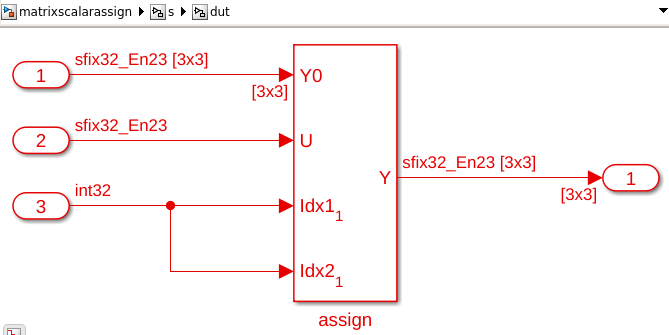produces this Verilog code snippet:

```always @* begin if ((In3 == 32'sb00000000000000000000000000000001) && (In3 == 32'sb00000000000000000000000000000001)) begin assign_out1 = In2; end else begin assign_out1 = In1; end if ((In3 == 32'sb00000000000000000000000000000010) && (In3 == 32'sb00000000000000000000000000000001)) begin assign_out1 = In2; end else begin assign_out1 = In1; end ... if ((In3 == 32'sb00000000000000000000000000000011) && (In3 == 32'sb00000000000000000000000000000011)) begin assign_out1 = In2; end else begin assign_out1 = In1; end end```
This Verilog code violates the check because even though the index values assigned of the matrix variable assign_out1 are different between the two cascaded `if` statements, the matrix variable is still being assigned multiple values in cascading conditional regions.

#### Selector Block

If a Selector block is in a model that generates HDL code, a violation of this check might occur.

For example, a model containing a Selector block in the DUT with the Selector block property Index Option having more than one dimension and set to `Index vector (port)`: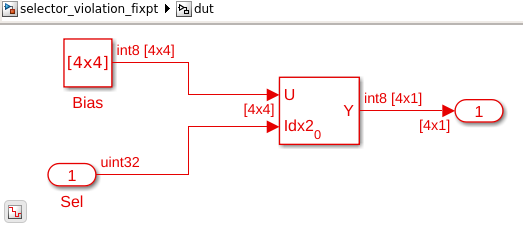produces this generated Verilog code that causes a violation by assigning the same variable Selector_out1 multiple values for each of its indices in multiple cascaded `if` statements.

```always @* begin Selector_out1 = Bias_out1; Selector_out1 = Bias_out1; Selector_out1 = Bias_out1; Selector_out1 = Bias_out1; if (Sel == 32'b00000000000000000000000000000010) begin Selector_out1 = Bias_out1; Selector_out1 = Bias_out1; Selector_out1 = Bias_out1; Selector_out1 = Bias_out1; end if (Sel == 32'b00000000000000000000000000000001) begin Selector_out1 = Bias_out1; Selector_out1 = Bias_out1; Selector_out1 = Bias_out1; Selector_out1 = Bias_out1; end if (Sel == 32'b00000000000000000000000000000000) begin Selector_out1 = Bias_out1; Selector_out1 = Bias_out1; Selector_out1 = Bias_out1; Selector_out1 = Bias_out1; end end```

#### Math Function Block Set as Reciprocal

If a Math Function block with the Function set as `reciprocal`, the Algorithm method set as `Exact`, and the Saturate on integer overflow selected as `on` is in a DUT in a model that generates HDL code, a violation of this check might occur.

For example, a model containing the Math Function block with the preceding settingsproduces this Verilog Code snippet that demonstrates the violation of a value assignment to the variable ufix_sameasinput_out1 in two parallel conditional `if` statements:

```always @(In1) begin ufix_sameasinput_div_temp = 16'b0000000000000000; if (In1 == 16'b0000000000000000) begin ufix_sameasinput_out1 = 16'b1111111111111111; end else begin ufix_sameasinput_div_temp = 13'b1000000000000 / In1; ufix_sameasinput_out1 = ufix_sameasinput_div_temp; end if (In1 == 16'b0000000000000000) begin ufix_sameasinput_out1 = 16'b1111111111111111; end end```